• 精通matlab傅里叶变换及逆变换和快速傅里叶变换
• 一幅图像经过傅里叶变换值周如何分别提取零级谱和正一级谱？</p>开发语言
• 图像的傅里叶变换，利用快速傅里叶进行图像的频率分析然后画出图形
• 中国科技论文在线 基于 MATLAB GUI 的傅里叶变换分析的仿 真设计 高恒伟宋余君何辉* 中国矿业大学信电学院江苏徐州 221008 摘要本文简要阐述了傅里叶变换的基本原理傅里叶变换的性质傅里叶变换的尺度变换 性频移特性...
• 今天，二狗给大家讲一讲Matlab实现傅里叶变换。大家都知道，信号分为两种，确定信号和不确定信号。在确定信号中，有两个非常重要的类别，时域分析和频域分析。而将两者充分结合的，就是我们今天要讲的傅里叶变换。绝...


嗨，大家好。二狗又回来了。今天，二狗给大家讲一讲Matlab实现傅里叶变换。大家都知道，信号分为两种，确定信号和不确定信号。在确定信号中，有两个非常重要的类别，时域分析和频域分析。而将两者充分结合的，就是我们今天要讲的傅里叶变换。绝大多数工科狗在大一或者大二的时候，都或多或少接触过傅里叶变换。二狗也不例外。当初二狗学《复变函数与积分变换》时，差点被搞成死狗，就是因为傅里叶变换。下面这个三维图就是频域和时域上的周期函数，非常形象的显示了这个道理。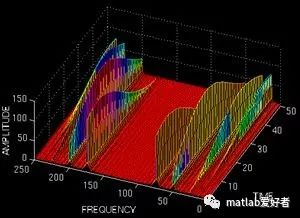由上可知，在空间中。从频率或者时域的角度都可以描述这个周期函数。下面，我们先帮助大家简单回忆一下傅里叶变换的基本原理。周期函数都是时域表达式，既然有周期，当然也就有频率。有些时候，我们需要用到函数的频域表达式。这就需要在频域和时域之间转化。最简单的就是利用周期函数的三角函数展开式，公式如下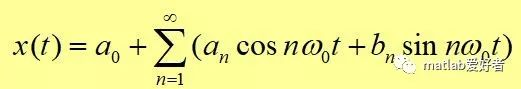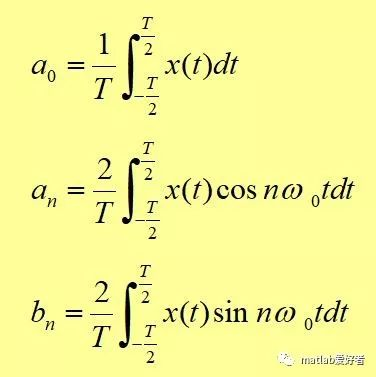以上是三角展开形式，今天我们不用这个，完全是为了方便大家理解，所以先说了一个简单的(见谅)。我们今天要用的是复指数形式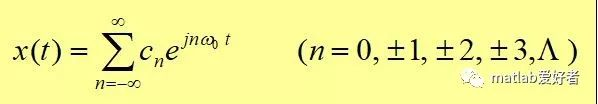当然，两种形式可以相互转换的，只需要利用欧拉公式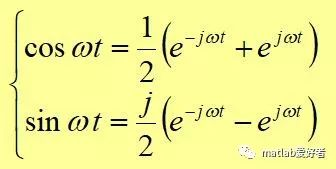下面，我举一个简单的函数作为例子f(t)=sin(t)+2*cos(t)。我们来对它进行傅里叶变换。根据已有的公式，我们一步步推进，我们先画出这个函数的图像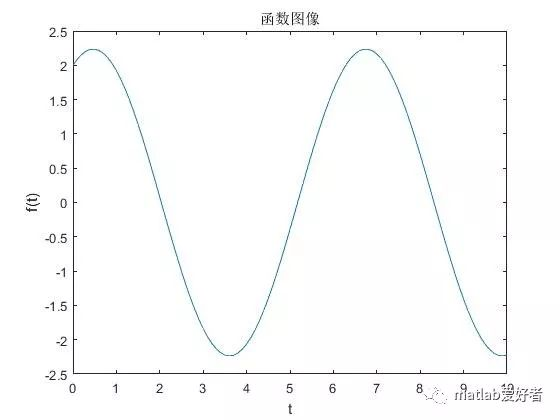下面的几步将傅里叶变换的参数全部求解出来，依据的就是复指数形式表达式(看不懂的同学倒回去看一看刚才讲的公式)。k=-N:N;W=pi*k/(N*dt);Fw=dt*ft*exp(-j*t1'*W);然后，对Fw进行一次绝对值变化。得到最终的结果。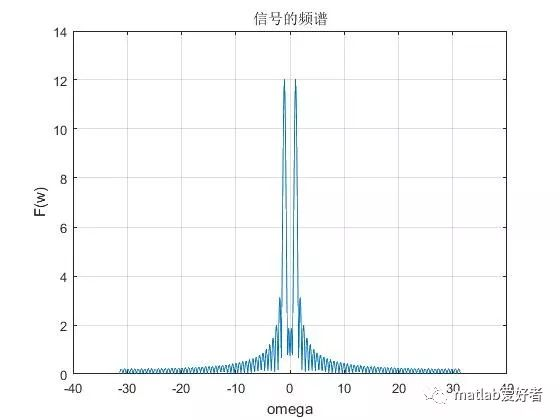以上就是信号的频域分析。即傅里叶表达式图像既然有频域函数，那当然就有频谱图。周期函数往往是连续的，但是频率却是离散的。废话不说了，上代码：Ts=0.4;  %采样周期,可通过改变采样周期大小观察抽样信号的频谱变化dt=0.1;t1=0:dt:10;ft=sin(t1)+2*cos(t1);%subplot(1)plot(t1,ft);%gridaxis('auto');xlabel('t');ylabel('f(t)');title('函数图像');hold onfigureN=400;k=-N:N;W=pi*k/(N*dt);Fw=dt*ft*exp(-j*t1'*W);plot(W,abs(Fw));grid;axis('auto')xlabel('omega');ylabel('F(w)');title('信号的频谱');hold onfigure%下面代表抽样过程t2=0:Ts:10;fst=sin(t2)+2*cos(t2);plot(t1,ft,'r*');hold onfigure%stem适合画火柴棍型图stem(t2,fst);gridaxis('auto')xlabel('t');ylabel('fs(t)');title('抽样后的信号');%hold offFsw=Ts*fst*exp(-j*t2'*W);%subplot(224)plot(W,abs(Fsw));gridaxis('auto')xlabel('omega');ylabel('Fs(w)');title('抽样信号的频谱');

展开全文• 三课时精通matlab傅里叶变换及逆变换和快速傅里叶变换 图像和算法等领域有...三课时精通matlab傅里叶变换及逆变换和快速傅里叶变换

图像和算法等领域有多年研究和项目经验；指导发表科技核心期刊经验丰富；多次指导数学建模爱好者参赛。宋星星

¥12.00

立即订阅

扫码下载「CSDN程序员学院APP」，1000+技术好课免费看
APP订阅课程，领取优惠，最少立减5元 ↓↓↓订阅后：请点击此处观看视频课程

视频教程-三课时精通matlab傅里叶变换及逆变换和快速傅里叶变换-Matlab

学习有效期：永久观看

学习时长：22分钟

学习计划：1天

难度：低

「口碑讲师带队学习，让你的问题不过夜」讲师姓名：宋星星

工程师

讲师介绍：图像和算法等领域有多年研究和项目经验；指导发表科技核心期刊经验丰富；多次指导数学建模爱好者参赛。

☛点击立即跟老师学习☚

「你将学到什么？」

精通matlab傅里叶变换及逆变换和快速傅里叶变换

「课程学习目录」

1.精通matlab傅里叶变换

2.精通matlab傅里叶逆变换

3.精通matlab快速傅里叶变换

「7项超值权益，保障学习质量」

大咖讲解

技术专家系统讲解传授编程思路与实战。

答疑服务

专属社群随时沟通与讲师答疑，扫清学习障碍，自学编程不再难。

课程资料+课件

超实用资料，覆盖核心知识，关键编程技能，方便练习巩固。（部分讲师考虑到版权问题，暂未上传附件，敬请谅解）

常用开发实战

企业常见开发实战案例，带你掌握Python在工作中的不同运用场景。

大牛技术大会视频

2019Python开发者大会视频免费观看，送你一个近距离感受互联网大佬的机会。

APP+PC随时随地学习

满足不同场景，开发编程语言系统学习需求，不受空间、地域限制。

「什么样的技术人适合学习？」

想进入互联网技术行业，但是面对多门编程语言不知如何选择，0基础的你
掌握开发、编程技术单一、冷门，迫切希望能够转型的你
想进入大厂，但是编程经验不够丰富，没有竞争力，程序员找工作难。

「悉心打造精品好课，1天学到大牛3年项目经验」

【完善的技术体系】

技术成长循序渐进，帮助用户轻松掌握

掌握Matlab知识，扎实编码能力

【清晰的课程脉络】

浓缩大牛多年经验，全方位构建出系统化的技术知识脉络，同时注重实战操作。

【仿佛在大厂实习般的课程设计】

课程内容全面提升技术能力，系统学习大厂技术方法论，可复用在日后工作中。

「你可以收获什么？」

熟练matlab傅里叶变换及逆变换和快速傅里叶变换

掌握matlab傅里叶变换及逆变换和快速傅里叶变换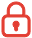订阅课程 开始学习


展开全文• ## MATLAB傅里叶变换

千次阅读 2015-11-19 19:36:10
title('lena傅里叶变换') subplot(3,2,3); imshow(I2); title('Peppers原图') fftI2=fft2(I2); %二维离散傅立叶变换 sfftI2=fftshift(fftI2); %直流分量移到频谱中心 RR2=real(sfftI2); %取傅立叶变换的实部 II2...
function [ output_args ] = Untitled2( input_args )
%读入原图像文件
%读入原图像文件
subplot(3,2,1);
imshow(I1);
title('lena原图')
fftI1=fft2(I1);
%二维离散傅立叶变换
sfftI1=fftshift(fftI1);
%直流分量移到频谱中心
RR1=real(sfftI1);
%取傅立叶变换的实部
II1=imag(sfftI1);
%取傅立叶变换的虚部
A1=sqrt(RR1.^2+II1.^2);
%计算频谱幅值
A1=(A1-min(min(A1)))/(max(max(A1))-min(min(A1)))*225;
%归一化
subplot(3,2,2);
imshow(A1);
title('lena傅里叶变换')
subplot(3,2,3);
imshow(I2);
title('Peppers原图')
fftI2=fft2(I2);
%二维离散傅立叶变换
sfftI2=fftshift(fftI2);
%直流分量移到频谱中心
RR2=real(sfftI2);
%取傅立叶变换的实部
II2=imag(sfftI2);
%取傅立叶变换的虚部
A2=sqrt(RR2.^2+II2.^2);
%计算频谱幅值
A2=(A2-min(min(A2)))/(max(max(A2))-min(min(A2)))*225;
%归一化
subplot(3,2,4);
imshow(A2);
title('Peppers傅里叶变换')

end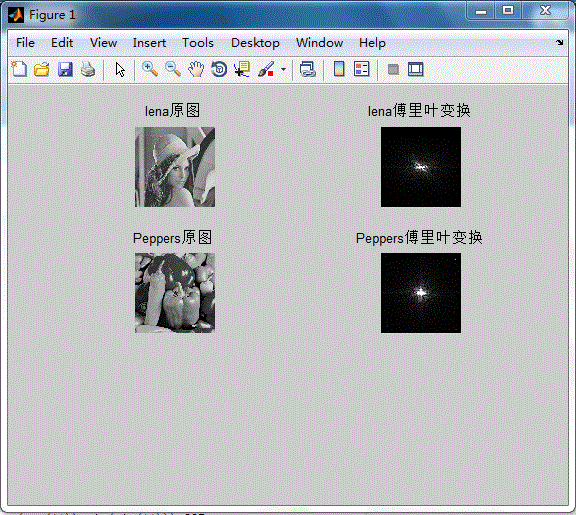展开全文函数
• Matlab 傅里叶变换模和相位和各种滤波处理 傅里叶变换模和相位 img=imread'h:\img.png; f=fft2(img; %傅里叶变换 f=fftshift(f; %使图像对称 r=real(f; %图像频域实部 i=imag(f; %图像频域虚部 margin=log(abs(f; %...
• matlab傅里叶变换实现滤波器 高通、低通 以及多种滤波因子
• 附 页 班级 信工142 学号 22 姓名 何岩 ...求信号的离散时间傅立叶变换并分析其周期性和对称性 给定正弦信号x(t)=2*cos(2*pi*10*t,fs=100HZ,求其DTFT (a)代码 f=10;T=1/f;w=-10:0.2:10; t1=0:0.0001:1;t2=0:0.01:1; n
• 基于matlab傅里叶变换与反变换的标准形式。
• 傅里叶变换与信号频谱图MATLAB 仿真实验傅里叶变换与信号频谱图本实验将简要介绍如何利用FFT 函数描绘指定信号的频谱图像。一、相关函数1、FFT 函数离散傅里叶(Fourier )变换函数。【语法】Y = fft(X)Y = fft(X,n)Y ...
• 运用傅里叶变换对信号进行简单的滤波原理将信号进行傅里叶变换可以信号中有哪些频率成分，将需要滤除的频率成分的幅值置零，然后进行傅里叶逆变换就可以达到滤波的目的。注意点运行FFT进行变换时需要考虑奈奎斯特...
• 自己写的傅里叶变换函数，带绘图，时域、频域对比。 输入参数data，时域信号。 Fs采样频率需根据实际修改。
• matlab编程写出的傅里叶变换的代码，用的是矩阵的内积和for循环这两种方法
• matlab中，利用傅里叶变换对图像进行处理，实现图像锐化功能。代码
• 满意答案fangong19782013.09.10采纳率：56%等级：...下面介绍一下用法格式:[tfr, t, f] = tfrstft(x) % 计算时间序列x的短时傅里叶变换，参数tfr为短时傅里叶变换系数，t为系数tfr对应的时刻，f为归一化频率向量[tfr...
• MATLAB傅里叶变换后寻找频谱次大值对应的位置 第一篇 data_double=transpose(x); N=length(data_double);n=0:N-1; y=fft(data_double,N);m=abs(y);f1=n*fs/N; figure; plot(f1,m); [pks,locs]=max(m); f1(locs) ...
• clc clear fs=10000; % 采样频率>2倍的信号频率 N=256000; % 采样点数目（=2的幂次） n=0:N-1; % 构造采样点序列 t=n/fs; % 得到采样时间序列，t=nT=n/fs A=1sin(2pi300t);%载波 B=0.1sin(2pi15t);... % FFT
• 另个就fft函数本身 暂不分析 关于x的修改 在x的赋值行后加入下列语句，得到了一定程度上的想象中的东西，对结果看不懂，和我在纸上写的傅里叶变换结果不一样，这里转折下去看下这东西怎么玩的吧 for j = 1:2*Fs x(j......

# matlab傅里叶变换matlab 订阅